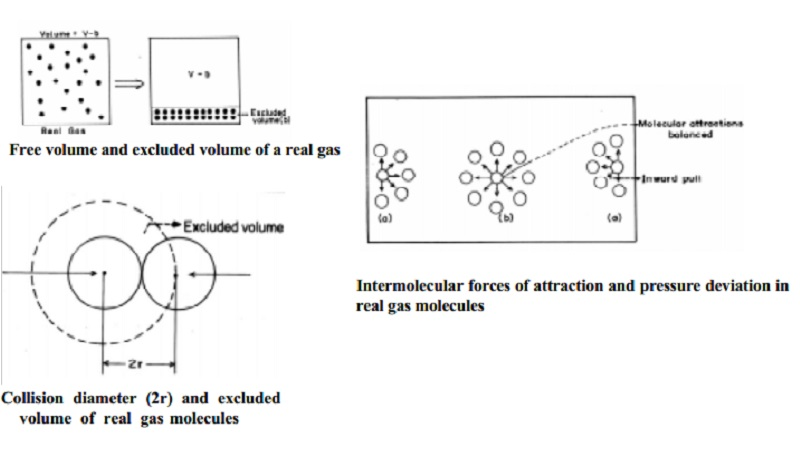Home | | Chemistry | Vanderwaal's Equation of state : Units, Significance, Limitations

# Vanderwaal's Equation of state : Units, Significance, LimitationsFor an ideal gas PV = nRT, is considered as the equation of state. By including the correction terms in the ideal gas equation to account for

Vanderwaal's Equation of state

For an ideal gas PV = nRT, is considered as the equation of state. By including the correction terms in the ideal gas equation to account for

(i) volume of the gaseous molecules themselves in V and (ii) the intermolecular forces of attraction in pressure, P, the equation of state for the real gas is arrived, J.O Vanderwaal's deduced the equation of state of real gases.

i)  Volume correction of real gas

The volume of a gas is the free space in the container in which molecules move about. Volume V of an ideal gas is the same as the volume of the container. The volume of a real gas is, therefore, ideal volume minus the volume occupied by the gas molecules themselves. If Vm is the volume of the single molecule then, the excluded volume which is termed as "b" is determined as follows.Let us consider two colliding molecules with radius `r'. The space indicated by dotted sphere of radius 2r will not be available for other molecules to freely move about. (i.e) the dotted spherical volume is known as excluded volume per pair of the molecules.

Thus,

excluded volume for two molecules = 4/3 x pi (2r) 3

=8( 4/3 pi r 3 )

Excluded volume per molecule

VC = � x 8 x 4/3 x pi r 3

= 4 Vm

where Vm is the actual volume of a single molecule.

The excluded volume for n molecules, 'b' = 4n Vm, where 4 Vm is the excluded volume of a molecule.

The corrected volume of the real gas is = (V-b) = free space for molecular movement.

ii) Pressure Correction

In a real gas the pressure deviation is caused by the intermolecular forces of attraction. According to kinetic theory, the pressure of the gas is directly proportional to forces of bombardment of the molecules on the walls of the container. Consider a molecule placed in the interior of the container. It is surrounded equally by other gas molecules in all directions such that the forces of attraction in any direction is cancelled (or) nullified by similar force operating in the opposite direction. However a molecule near the wall of the container which is about to strike is surrounded unequally by other gaseous molecules as shown in Fig.

The molecule near the wall experiences attractive forces only such that it will strike the wall with a lower force which will exert a lower pressure than if such attractive forces are not operating on it. Therefore it is necessary to add the pressure correction term to the pressure of the gas to get the ideal pressure. The corrected pressure should be P + p' where p' is the pressure correction factor.The force of attraction experienced by a molecule near the wall depends upon the number of molecules per unit volume of the bulk of the gas. It is found experimentally that, p' is directly proportional to the square of the density of the gas (r) which is a measure of the intermolecular attraction of the molecules.

P� = p2

Density is inversely related to the volume `V' which is the volume occupied by one mole of the gas. Therefore P of one mole of the gas is given by

P� = 1/ V2

where `a' is a proportionality constant that depends upon the nature of the gas.

corrected pressure   =          P + P

= P + (a/ V2)

Replacing the term for corrected volume and the corrected pressure in the ideal gas equation for one mole, the equation of state of the real gas is

(  P + (a/ V2) ) + ( V-b ) =  RT

where `a' and `b' are known as vanderwaal's constants.

This equation is also known as Vanderwaal's equation of state.

If there are `n' moles of the real gas then the vanderwall's equation becomes

P + (n2a / V2) = (V-nb) = nRT

Units for vanderwaal's constant

The dimensions of the vanderwaal's constant a and b depend upon the units of P and V respectively.

a= Pressure . (Volume)2 / mole2

a  =  atm . dm6 mol-2 (_ 1 litre = 1 dm3)

Thus a is expressed as atm.dm6 mol-2 units. If volume is expressed in dm3 then b is expressed as

Vo1/n = dm3/mol

V/ n =dm3mol-1

Unit of b   = dm3mol-1

Significance of Vanderwaal's constant (a) and (b)

1.     The term a/V2 is the measure of the attractive forces of the molecules. It is also called as the cohesion pressure (or) internal pressure.

2.     The inversion temperature of a gas can be expressed in terms of `a' and `b'

Ti= 2a / Rb

3.     The vanderwaal constants `a' and `b' enable the calculation of critical constants of a gas.

Limitations of Vanderwaal's equation

1.     It could not explain the quantitative aspect of deviation satisfactorily as it could explain the qualitative aspects of P and V deviations.

2.     The values of `a' and `b' are also found to vary with P and T, and such variations are not considered in the derivation of Vanderwaal equation.

3.     Critical constants calculated from Vanderwaal's equation deviate from the original values determined by other experiments.

Study Material, Lecturing Notes, Assignment, Reference, Wiki description explanation, brief detail
11th 12th std standard Class Organic Inorganic Physical Chemistry Higher secondary school College Notes : Vanderwaal's Equation of state : Units, Significance, Limitations |